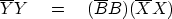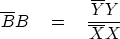Next: Crosscorrelation Up: CORRELATION AND SPECTRA Previous: Time-domain conjugate

## Spectral transfer function

Filters are often used to change the spectra of given data. With input X(Z), filters B(Z), and output Y(Z), we have Y(Z) = B(Z)X(Z) and the Fourier conjugate. Multiplying these two relations together, we getwhich says that the spectrum of the input times the spectrum of the filter equals the spectrum of the output. Filters are often characterized by the shape of their spectra; this shape is the same as the spectral ratio of the output over the input:(47)Next: Crosscorrelation Up: CORRELATION AND SPECTRA Previous: Time-domain conjugate
Stanford Exploration Project
10/21/1998